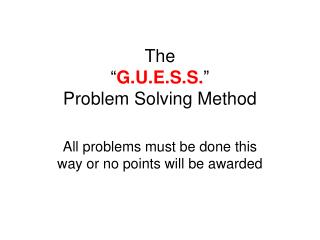# The “ G.U.E.S.S. ” Problem Solving Method - PowerPoint PPT PresentationDownload PresentationThe “ G.U.E.S.S. ” Problem Solving Method

The “ G.U.E.S.S. ” Problem Solving MethodDownload Presentation## The “ G.U.E.S.S. ” Problem Solving Method

- - - - - - - - - - - - - - - - - - - - - - - - - - - E N D - - - - - - - - - - - - - - - - - - - - - - - - - - -
##### Presentation Transcript

1. The “G.U.E.S.S.”Problem Solving Method All problems must be done this way or no points will be awarded

2. G.U.E.S.S. is an Acronym • G stands for given • U stands for unknown • E stands for equation • S stands for substitute • S stands for solve

3. G = Given • James drives 100 km in 5 hr what’s his speed? • What information is given in the problem? • The distance and the time • G d = 100 km t = 5 hr • Notice all values have a unit/label

4. U = Unknown • James drives 100 km in 5 hr what’s his speed? • What is the question, what are you solving for? • Speed • U S = ?

5. E = Equation • James drives 100 km in 5 hr what’s his speed? • What is the equation/formula that we use to solve for speed? • E s = d/t

6. S = Substitute • James drives 100 km in 5 hr what’s his speed? • G d = 100 km t = 5 hr • U s = ? • E s = d/t • Replace the variable with their value. • Replace d with 100 km and t with 5 hr • S s = 100 km/5 hr

7. S = Solve • James drives 100 km in 5 hr what’s his speed? • G d = 100 km t = 5 hr • U s = ? • E s = d/t • S s = 100 km/5 hr • S s = 20 km/hr • The solution includes a value with units

8. GUESS done horizontally • An alternative way

9. G = Given • James drives 100 km in 5 hr what’s his speed? • What information is given in the problem? • The distance and the time G d = 100 km t = 5 hr Notice all values have a unit/label

10. U = Unknown • James drives 100 km in 5 hr what’s his speed? • What is the question, what are you solving for? G U d = 100 km S = ? t = 5 hr

11. E = Equation • James drives 100 km in 5 hr what’s his speed? • What is the equation/formula that we use to solve for speed? G U E d = 100 km S = ? s = d/t t = 5 hr

12. S = Substitute • James drives 100 km in 5 hr what’s his speed? • Replace the variable with their value. • Replace d with 100 km and t with 5 hr G U E S d = 100 km S = ? s = d/t s = 100 km/5 hr t = 5 hr

13. S = Solve James drives 100 km in 5 hr what’s his speed? S S G U E d = 100 km S = ? s = d/t s = 20 km/hr s = 100 km/5 hr t = 5 hr

14. S = Solve James drives 100 km in 5 hr what’s his speed? S S G U E d = 100 km S = ? s = d/t s = 20 km/hr s = 100 km/5 hr t = 5 hr

15. Megan drives her scooter with an average speed of 23.0 km/h. How long will it take her to drive 9 km?

16. How far is it from school to the store if it takes 15 minutes to walk there, if you walk at 3 mi/h. Give your answer in km. G t = 15 min s = 3 mi/h U d=? km E s = d/t Before substituting convert min to h 15 min 1h 0.25 h 60 min x 0.25 h S 3 mi = d__ h 0.25 h 0.25 h x S d = 0.75 mi now convert mi to km d = 1.2 km 0.75 mi 1.609 km 1.2 km 1 mi